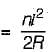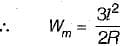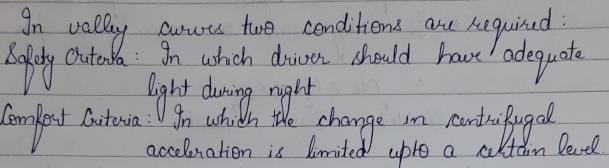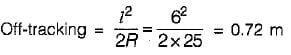# Test: Highway Geometric Design -2

## 10 Questions MCQ Test Topicwise Question Bank for Civil Engineering | Test: Highway Geometric Design -2

Description
Attempt Test: Highway Geometric Design -2 | 10 questions in 30 minutes | Mock test for Civil Engineering (CE) preparation | Free important questions MCQ to study Topicwise Question Bank for Civil Engineering for Civil Engineering (CE) Exam | Download free PDF with solutions
QUESTION: 1

### The mechanical extra widening required for 10.5 m wide pavement on a horizontal curve of radius R metre is given by  where l is the length of wheel base of vehicle in metres.

Solution:

Mechanical wideningwhere, n = no. of lanes
For 10.5 m wide pavement,
n=3QUESTION: 2

Solution:
QUESTION: 3

### The maximum design gradient for vertical profile of a road is

Solution:

Ruling gradient is the maximum gradient within, which the designer attempts to design the vertical profile of a road.

QUESTION: 4

On sag (or valley) curves the available sight distance is determined based on

Solution:QUESTION: 5

The maximum; width of a vehicle as recommended by IRC is

Solution:
QUESTION: 6

Transition curves are provided on the approach to horizontal curves in order to

Solution:
QUESTION: 7

Transition curve is provided in horizontal alignment

Solution:
QUESTION: 8

The ideal form of curve for the summit curve is

Solution:

Vertical curve:

Vertical alignment is the elevation of the centerline of the road. It may be in the form of a circular arc or a parabola

The vertical curve is divided into two categories

1) Summit curve:

It is a crest curve with convexity upward.

The deviation angle between the two interacting gradients is equal o the algebraic difference between them.

When an ascending gradient meets with descending gradients the deviation angle will be maximum

N = n1 – (-n2) = n1 + n2

Ideal shape of summit curve is circular.

But Square parabola is the best shape of the summit curve due to good riding qualities, simplicity of calculation, and uniform rate of change of grade.

2) Valley curve:

The maximum possible deviation angle is obtained when a descending gradient meets with an ascending gradient.

The overtaking sight distance is not included in the valley curve.

The cubic parabola is generally preferred.

The headlight sight distance available at the valley curve should be at least equal to the stopping sight distance.

QUESTION: 9

The off-tracking of a vehicle having a wheel base of 6.0 m and negotiating a curved path of mean radius 25 m is

Solution:QUESTION: 10

The important factor considered in the design of summit curves on highways is

Solution:

When a fast moving vehicle travels along a summit curve, the centrifugal force will act upwards, against gravity and hence a part of the pressure on the tyres and spring of the vehicle suspensions in relieved. So there is no problem of discomfort to passengers on summit curves, particularly because the deviation angles on roads are quite small. The only problem is designing summit curves is to provide adequate sight distances. The stopping sight distance or the absolute minimum sight distance should invariably be provided at all sections of the road system and so aiso on summit curves.Use Code STAYHOME200 and get INR 200 additional OFF Use Coupon Code